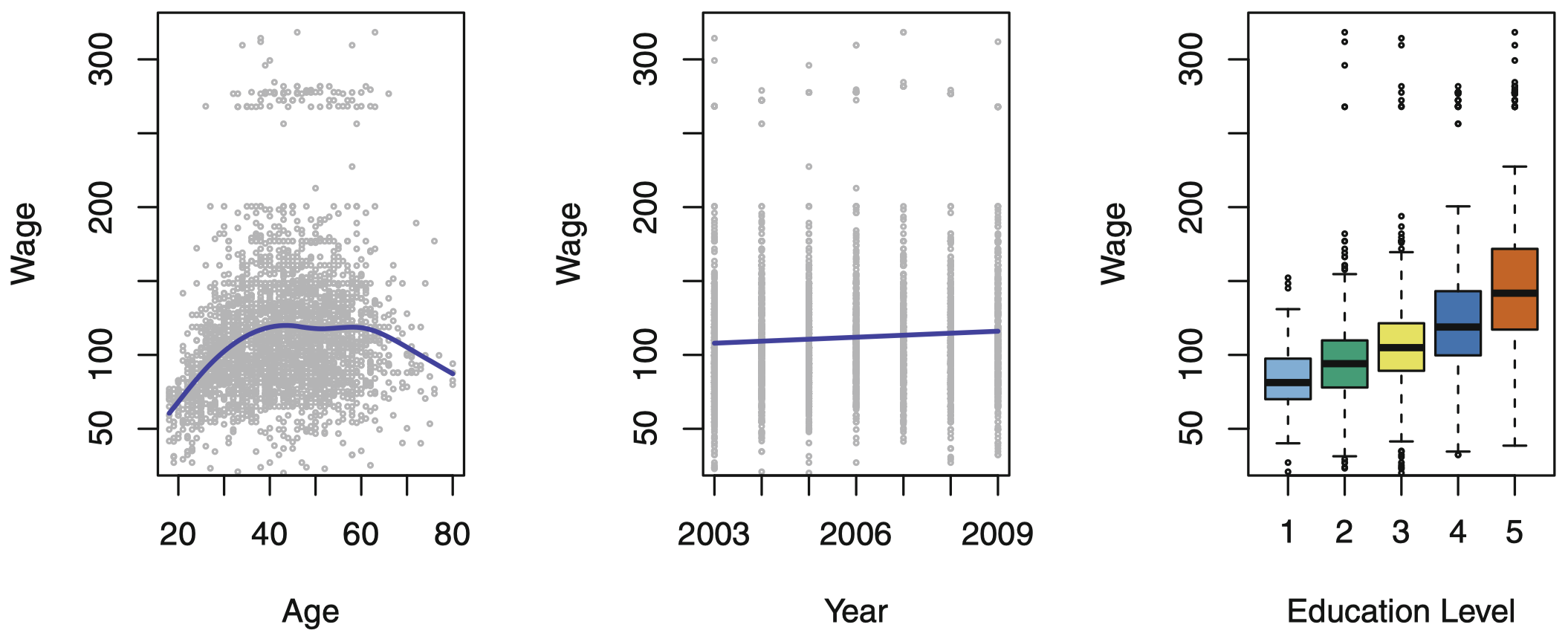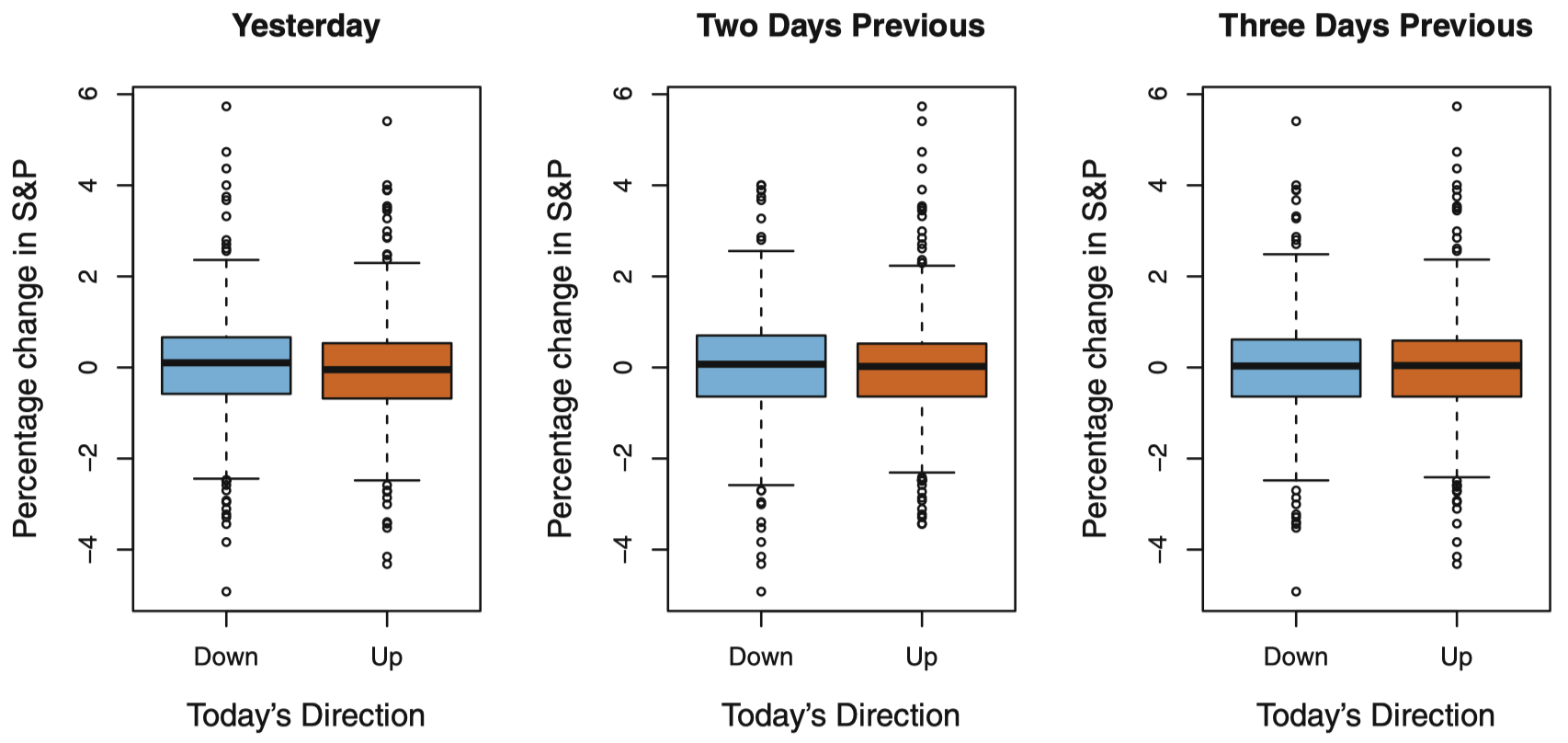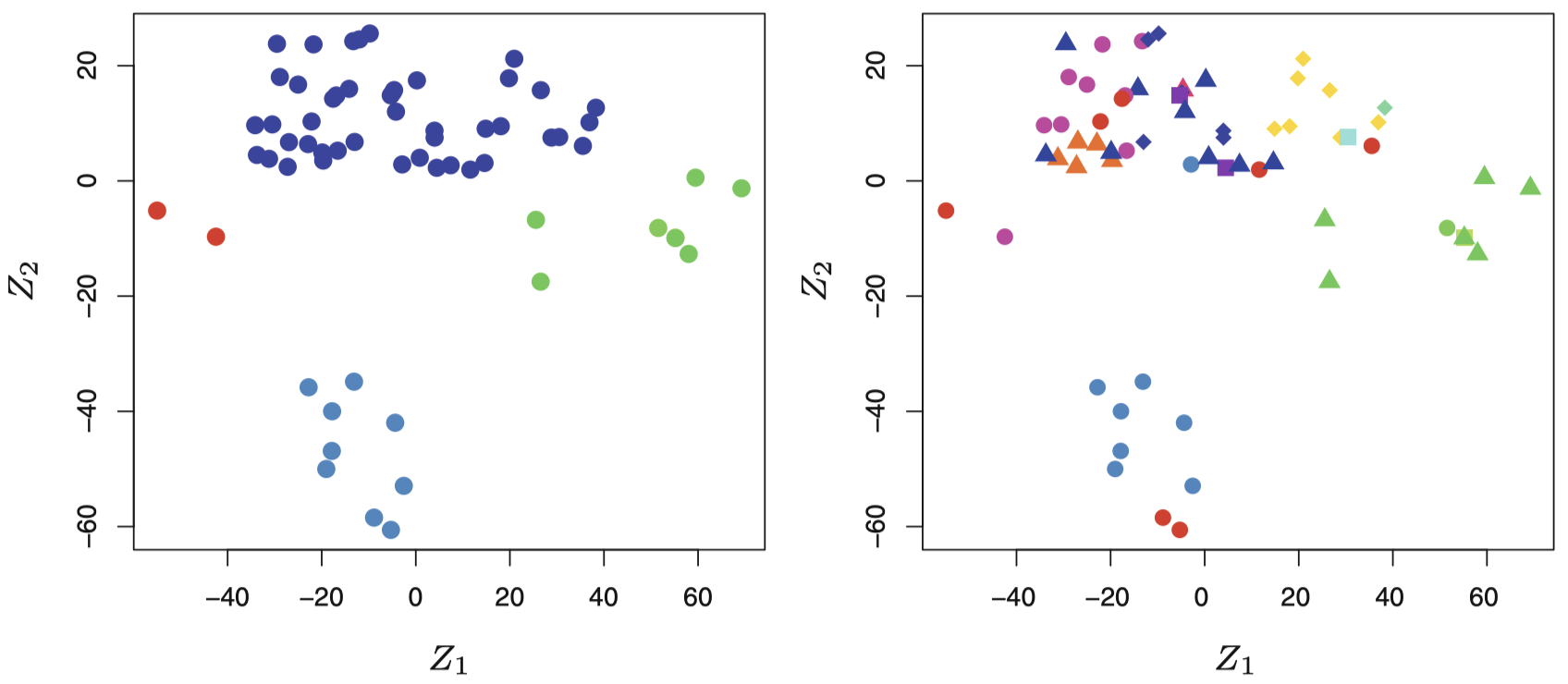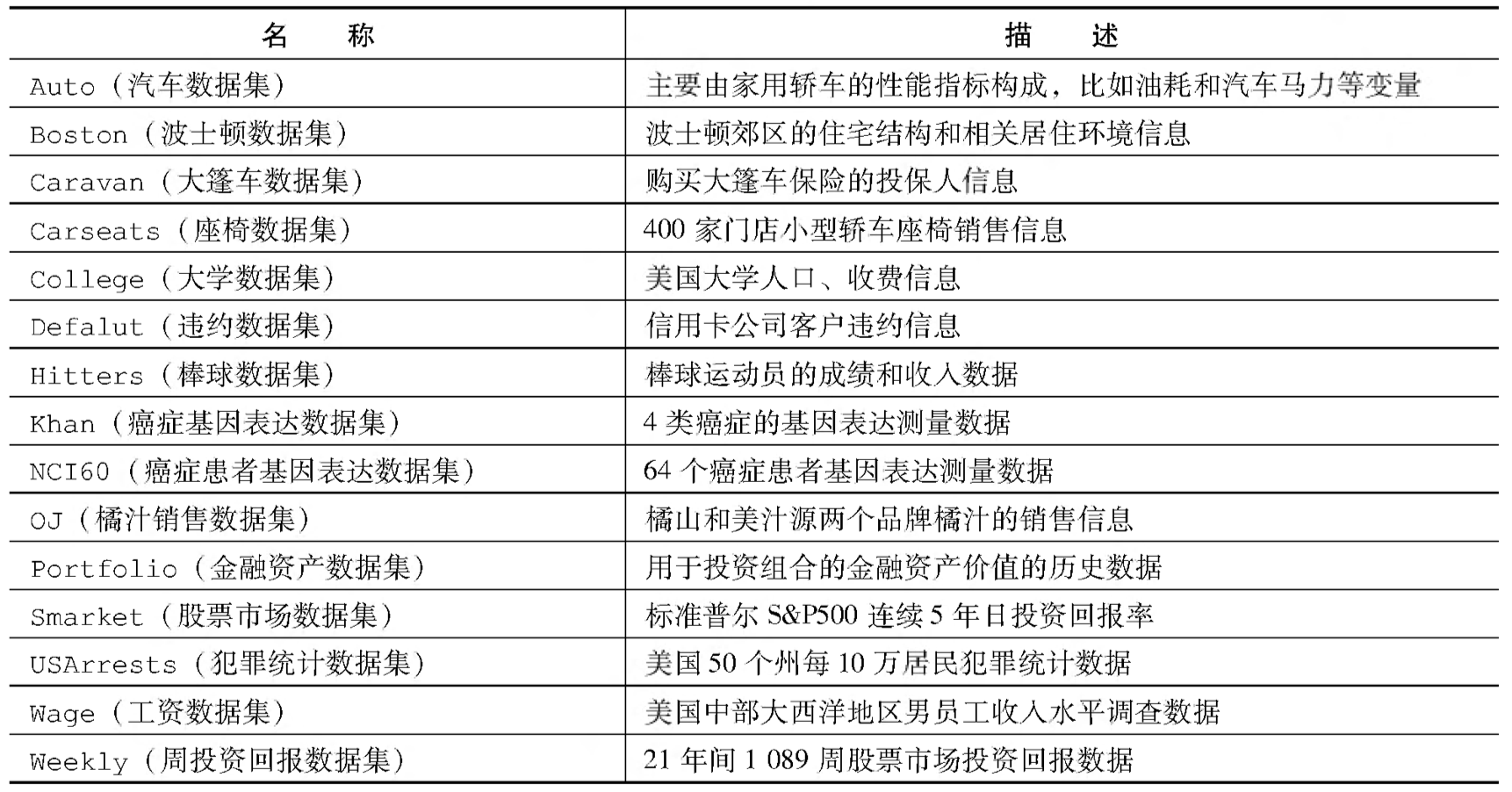# 计算统计学与数据科学 01：导论

## 墨尔本大学 MAST90083 课程笔记

Posted by YEY on August 3, 2020

# Lecture 01 导论

• Gareth, J., Daniela, W., Trevor, H., & Robert, T. (2013). An intruduction to statistical learning: with applications in R. Spinger.
• Hastie, T., Tibshirani, R., & Friedman, J. (2009). The elements of statistical learning: data mining, inference, and prediction. Spinger Science & Business Media.

## 1. 导论

### 1.1 内容安排

• 回归与分类
• 重采样和模型选择
• 样条 (Splines) 和 广义加性模型 (GAMs)
• 基于树的方法和 SVMs
• 无监督学习
• 神经网络

### 1.3 有监督学习：回归与分类

• 像工资数据这类涉及预测连续或定量的输出值。这通常称为 回归 (regression) 问题。
• 但是，在某些情况下，我们可能希望预测的是一个非数值的值，即一个类别或定性输出。这称为 分类 (classification) 问题。例如：股市数据。

• 输入 (特征)age, yeareducation
• 输出 (标签)wage• 输入 (特征)：过去 3 天的标准普尔指数 (S&P Index) 的变动比例
• 输出 (标签)Up (上涨) 或者 Down (下跌)### 1.4 无监督学习：聚类## 2. 表示方法

$n$：不同数据点或样本观测的个数。

$p$：用于预测的变量个数。

$\mathbf{X}=\begin{pmatrix} x_{11} & x_{12} & \cdots & x_{1p} \\ x_{21} & x_{22} & \cdots & x_{2p} \\ \vdots & \vdots & \ddots & \vdots \\ x_{n1} & x_{n2} & \cdots & x_{np} \\ \end{pmatrix}$

$x_i=\begin{pmatrix} x_{i1}\\ x_{i2}\\ \vdots\\ x_{ip}\\ \end{pmatrix}$

$\mathbf{x}_j=\begin{pmatrix} x_{1j}\\ x_{2j}\\ \vdots\\ x_{nj}\\ \end{pmatrix}$

$\mathbf{X}=(\mathbf{x}_1 \quad \mathbf{x}_2 \quad \cdots \quad \mathbf{x}_p)$

$\mathbf{X}=\begin{pmatrix} x_{1}^{\mathrm T}\\ x_{2}^{\mathrm T}\\ \vdots\\ x_{n}^{\mathrm T}\\ \end{pmatrix}$

$\mathbf{X}=\begin{pmatrix} x_{11} & x_{21} & \cdots & x_{n1} \\ x_{12} & x_{22} & \cdots & x_{n2} \\ \vdots & \vdots & & \vdots \\ x_{1p} & x_{2p} & \cdots & x_{np} \\ \end{pmatrix}$

$y_i$ 表示待预测的变量 (比如 wage) 的第 $i$ 个观测值。待预测变量的全部 $n$ 个观测值的集合，用如下向量表示：

$\mathbf{y}=\begin{pmatrix} y_{1}\\ y_{2}\\ \vdots\\ y_{n}\\ \end{pmatrix}$

• 向量：长度为 $n$，使用小写加粗字母表示，例如 $\mathbf{a}$。
• 向量：长度不为 $n$ (比如为特征数 $p$)，使用小写常规字母表示，例如 $a$。
• 标量：也用小写常规字母表示，例如 $a$。
• 矩阵：使用大写加粗字母表示，例如 $\mathbf{A}$。
• 随机变量：不论维数，一律使用大写常规字母表示，例如 $A$。

$\mathbf{A}=\begin{pmatrix} 1 & 2 \\ 3 & 4 \\ \end{pmatrix}, \quad \mathbf{B}=\begin{pmatrix} 5 & 6 \\ 7 & 8 \\ \end{pmatrix}$

$\mathbf{AB}=\begin{pmatrix} 1 & 2 \\ 3 & 4 \\ \end{pmatrix} \begin{pmatrix} 5 & 6 \\ 7 & 8 \\ \end{pmatrix}= \begin{pmatrix} 1\times 5+ 2\times 7 & 1\times 6+ 2\times 8 \\ 3\times 5+ 4\times 7 & 3\times 6+ 4\times 8 \\ \end{pmatrix}= \begin{pmatrix} 19 & 22 \\ 43 & 50 \\ \end{pmatrix}$

## 3. 主要内容和数据集

• 基本术语 (K 近邻分类器和一些相关问题)
• 线性回归
• 经典分类方法 (逻辑回归和线性判别分析)
• 交叉验证和 bootstrap (估计多种不同方法的准确性，以便选择最佳方法)
• 标准线性回归的潜在改进方式 (stepwise 选择、岭回归、主成分回归、偏最小二乘和 lasso)
• 非线性统计学习
• 基于树的方法，包括 bagging、boosting 和随机森林。
• 支持向量机
• 无监督学习：主成分分析、K 均值聚类和层次聚类
• 神经网络本作品采用知识共享署名-非商业性使用-相同方式共享 4.0 国际许可协议进行许可。 欢迎转载，并请注明来自：YEY 的博客 同时保持文章内容的完整和以上声明信息！